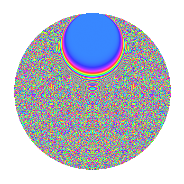# Properties

 Label 2009.2.cdLevel 2009 Weight 2 Character orbit cd Rep. character $$\chi_{2009}(4,\cdot)$$ Character field $$\Q(\zeta_{210})$$ Dimension 9312 Sturm bound 392

# Related objects

## Defining parameters

 Level: $$N$$ = $$2009 = 7^{2} \cdot 41$$ Weight: $$k$$ = $$2$$ Character orbit: $$[\chi]$$ = 2009.cd (of order $$210$$ and degree $$48$$) Character conductor: $$\operatorname{cond}(\chi)$$ = $$2009$$ Character field: $$\Q(\zeta_{210})$$ Sturm bound: $$392$$

## Dimensions

The following table gives the dimensions of various subspaces of $$M_{2}(2009, [\chi])$$.

Total New Old
Modular forms 9504 9504 0
Cusp forms 9312 9312 0
Eisenstein series 192 192 0

## Trace form

 $$9312q - 39q^{2} - 231q^{4} - 43q^{5} - 30q^{6} - 60q^{7} - 34q^{8} - 896q^{9} + O(q^{10})$$ $$9312q - 39q^{2} - 231q^{4} - 43q^{5} - 30q^{6} - 60q^{7} - 34q^{8} - 896q^{9} - 36q^{10} - 65q^{11} - 35q^{12} - 50q^{13} - 20q^{15} - 231q^{16} - 65q^{17} - 39q^{18} - 30q^{19} + 54q^{20} - 50q^{21} - 50q^{22} - 111q^{23} - 110q^{24} - 225q^{25} - 65q^{26} - 75q^{28} + 120q^{29} + 10q^{30} - 94q^{31} - 18q^{32} - 32q^{33} - 170q^{34} - 70q^{35} - 424q^{36} - 18q^{37} - 38q^{39} - 118q^{40} - 70q^{41} + 232q^{42} - 30q^{43} - 62q^{45} - 178q^{46} + 10q^{47} - 90q^{48} - 168q^{49} - 112q^{50} + 144q^{51} + 35q^{52} - 265q^{53} - 140q^{54} + 250q^{56} - 89q^{57} - 65q^{58} - 131q^{59} - 20q^{60} - 93q^{61} - 40q^{63} + 394q^{64} - 110q^{65} + 50q^{66} - 70q^{67} - 40q^{69} + 175q^{70} + 45q^{71} - 269q^{72} - 120q^{73} - q^{74} + 50q^{75} + 345q^{76} - 72q^{77} - 64q^{78} + 90q^{80} + 372q^{81} + 7q^{82} + 156q^{83} - 327q^{84} - 139q^{86} + 154q^{87} + 610q^{88} - 385q^{89} - 141q^{90} - 210q^{91} - 8q^{92} - 140q^{93} - 335q^{94} - 125q^{95} - 160q^{97} - 193q^{98} - 180q^{99} + O(q^{100})$$

## Decomposition of $$S_{2}^{\mathrm{new}}(2009, [\chi])$$ into irreducible Hecke orbits

The newforms in this space have not yet been added to the LMFDB.